Find the question about bathroom tiles, but don't go quackers!

# Area

This Math quiz is called 'Area' and it has been written by teachers to help you if you are studying the subject at high school. Playing educational quizzes is a user-friendly way to learn if you are in the 9th or 10th grade - aged 14 to 16.

It costs only \$12.50 per month to play this quiz and over 3,500 others that help you with your school work. You can subscribe on the page at Join Us

Area is all about how much space a flat shape takes up. See how well you can apply your knowledge of Area in this high school Math quiz.

A straight line has only one dimension, its length. Once we draw more lines in different directions to form an enclosed shape, we create something in 2 dimensions. In order to describe this we need more than just the length of each line, we also need to know how much space on a flat surface it takes up. This is known as its area, and we include the word ‘square’ in its units, such as cm2 (square centimeters) and m2 (square meters).

To be successful when solving area problems, you need to know a few formulae. The most common ones are for the rectangle (and therefore the square too), the triangle and the circle.

Complex shapes are made by combining these basic shapes, so be prepared to draw diagrams and split a shape up into rectangles and triangles. This may mean you have to work out some missing lengths.

In triangle problems, you may not know one of the perpendicular heights, but instead be given the hypotenuse. Don’t fall into the trap of using that number in your area calculation – you need to apply Pythagoras’ Theorem first, to find the shorter side.

A common type of question used in exams involves tiling an area. Usually the tiles’ dimensions are given in cm, while the area to be tiled is given in m2. You need to convert the tile lengths to meters, before working out the area of each tile. Remember to apply a common sense check to your final answer – if it doesn’t look like a reasonable answer, you have probably made a mistake with your units!

Question 1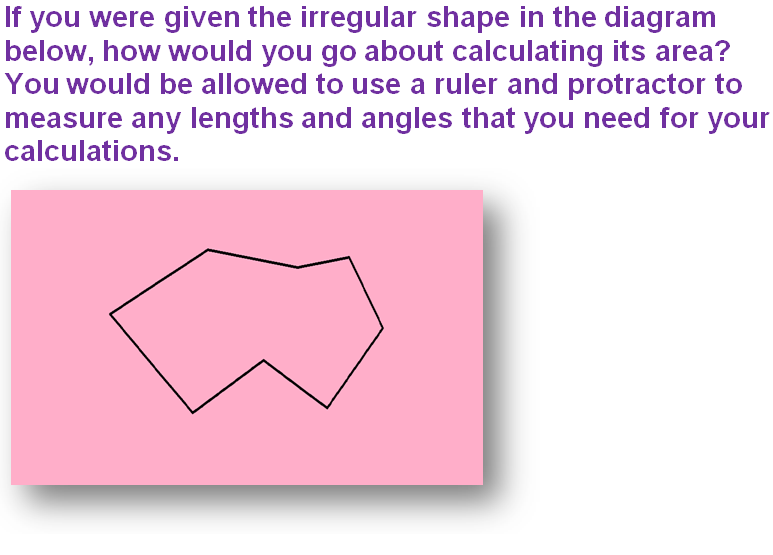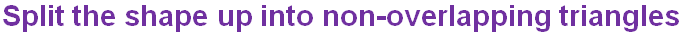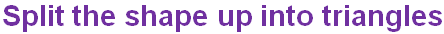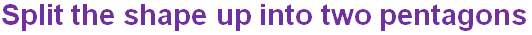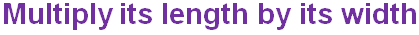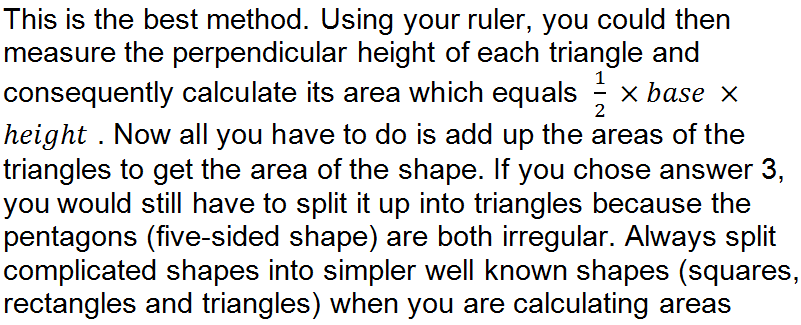Question 2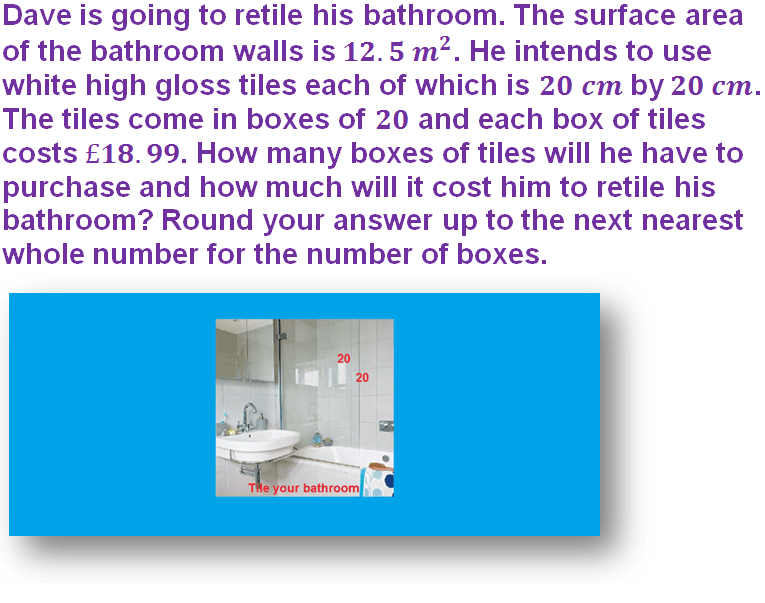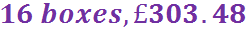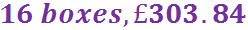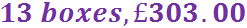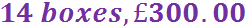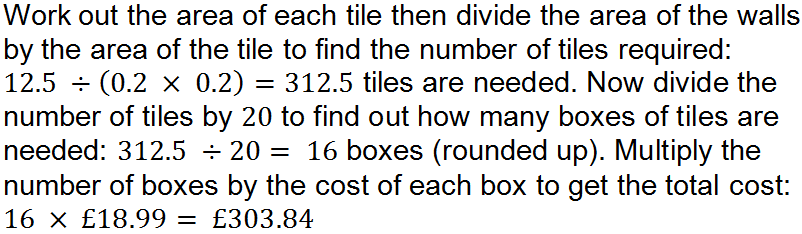Question 3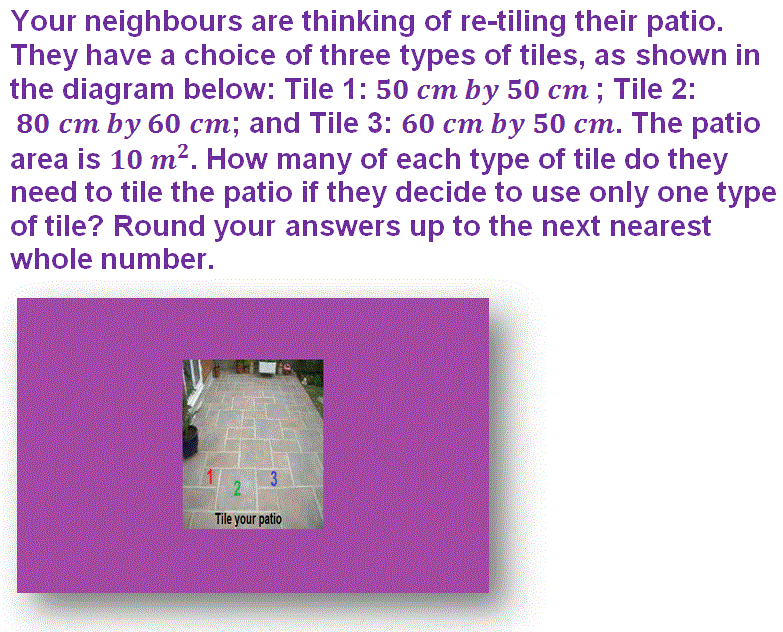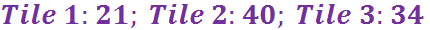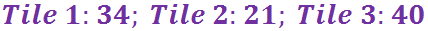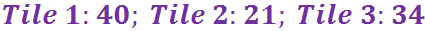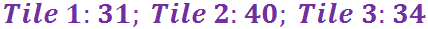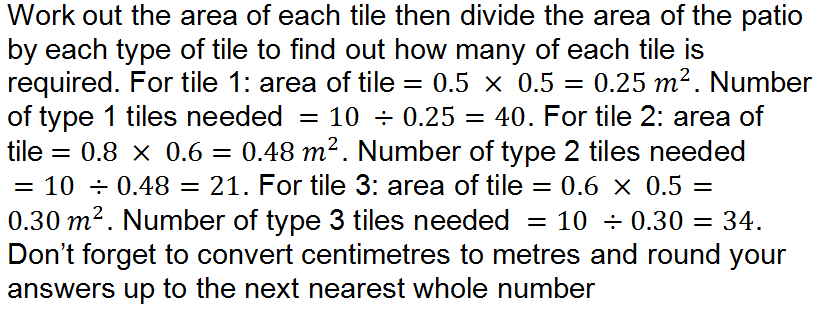Question 4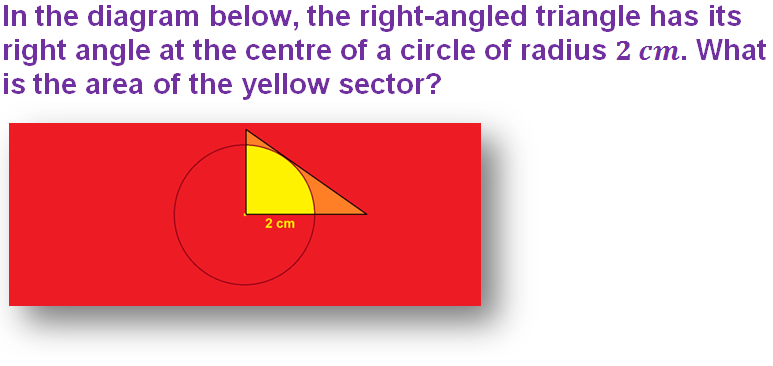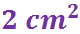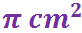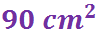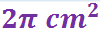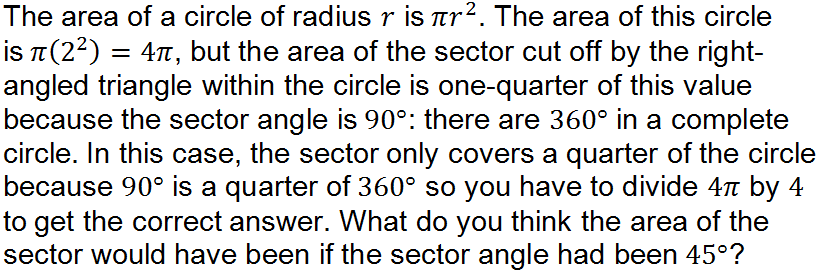Question 5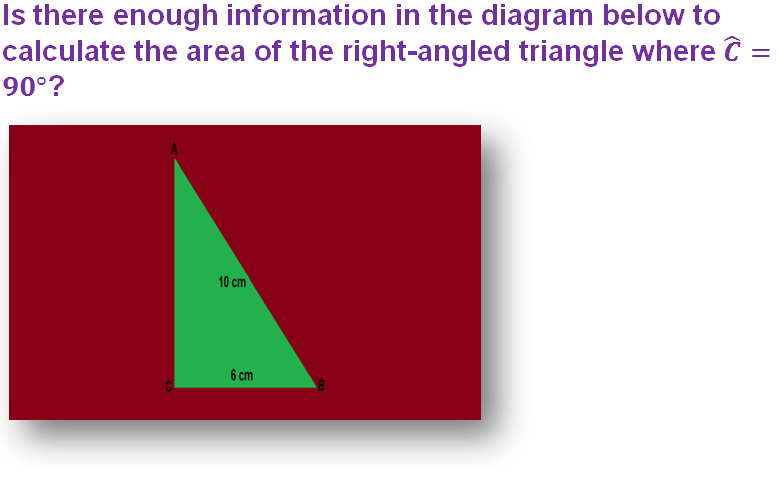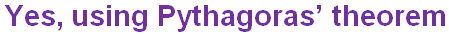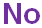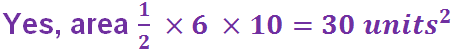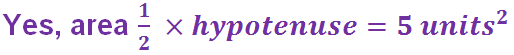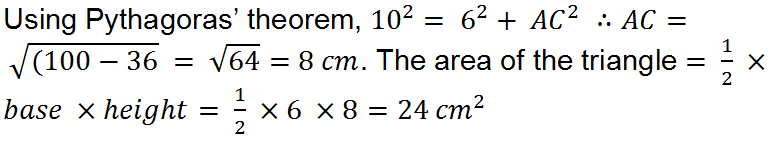Question 6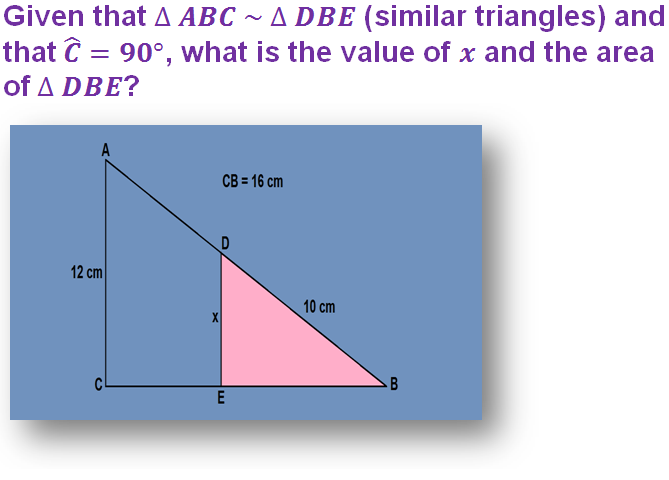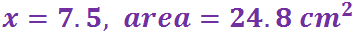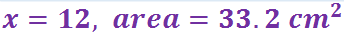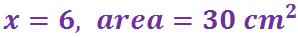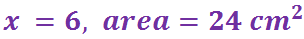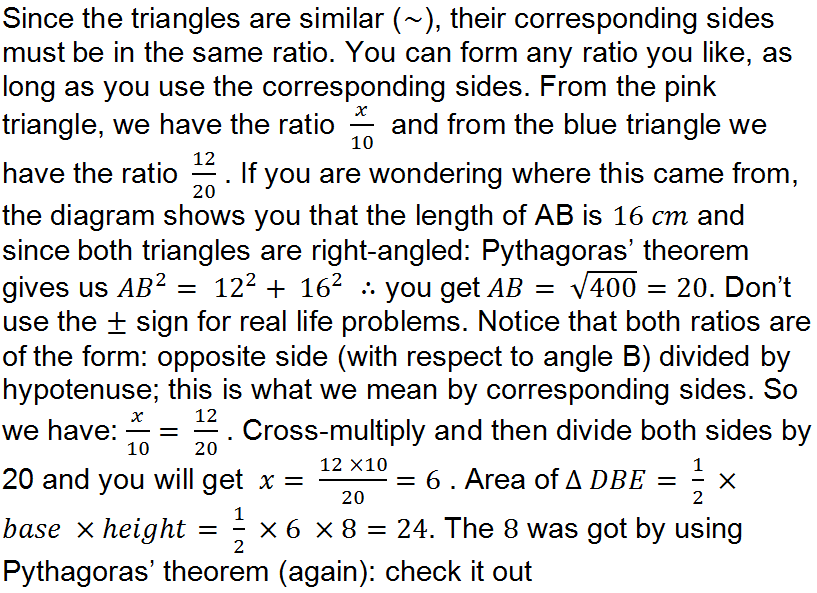Question 7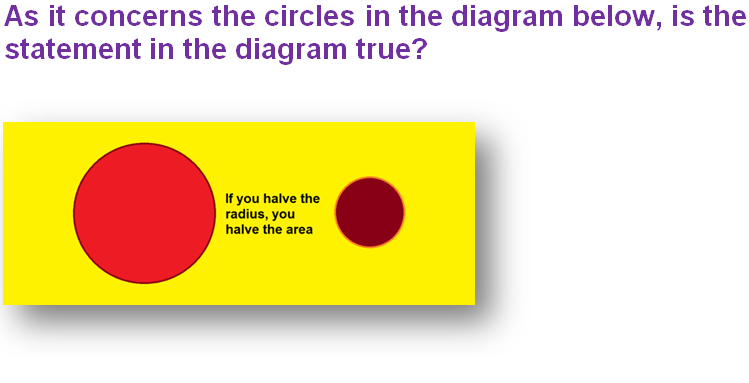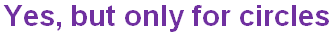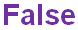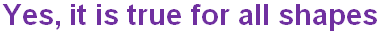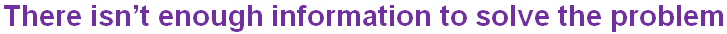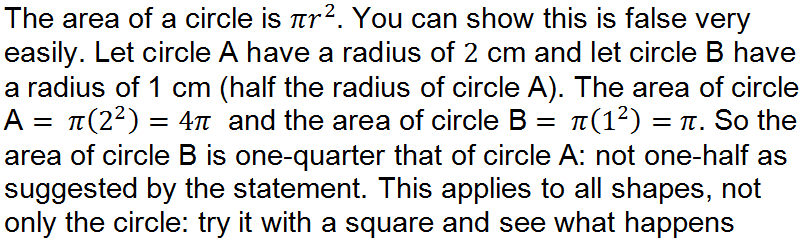Question 8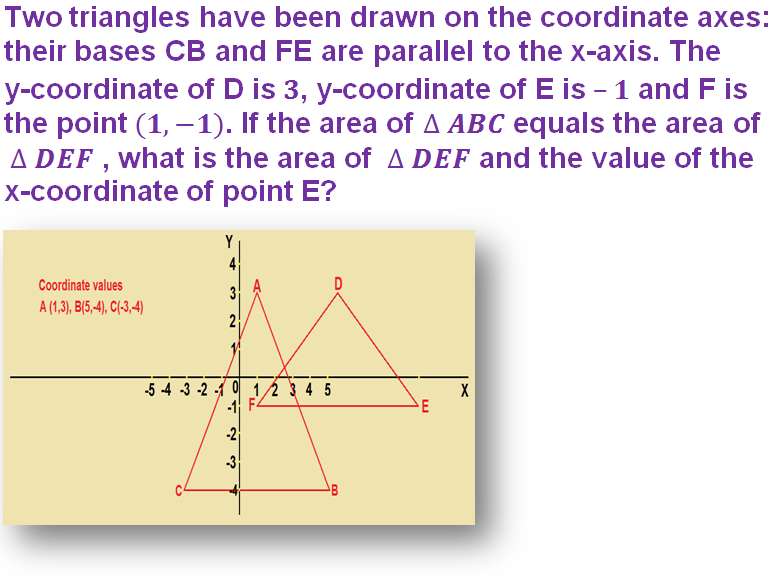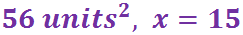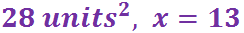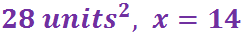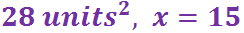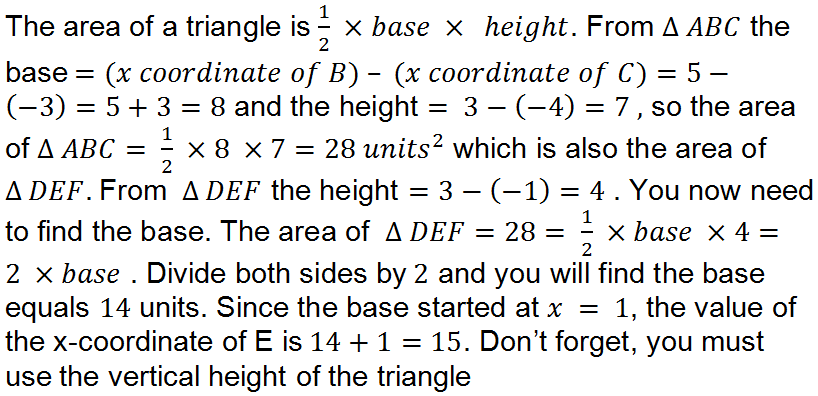Question 9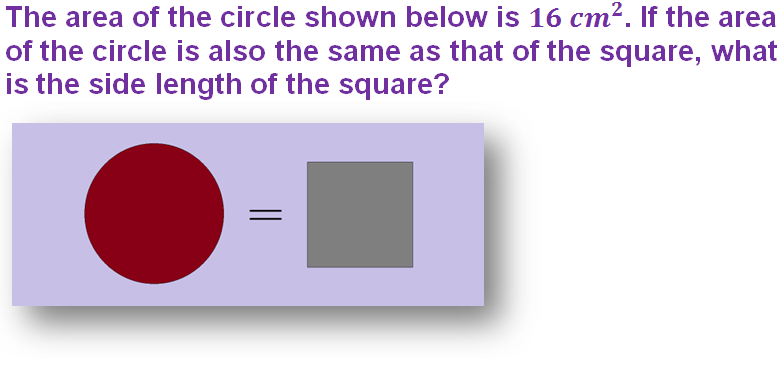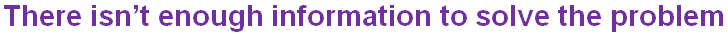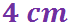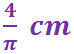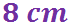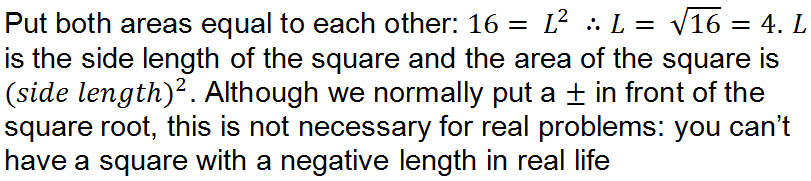Question 10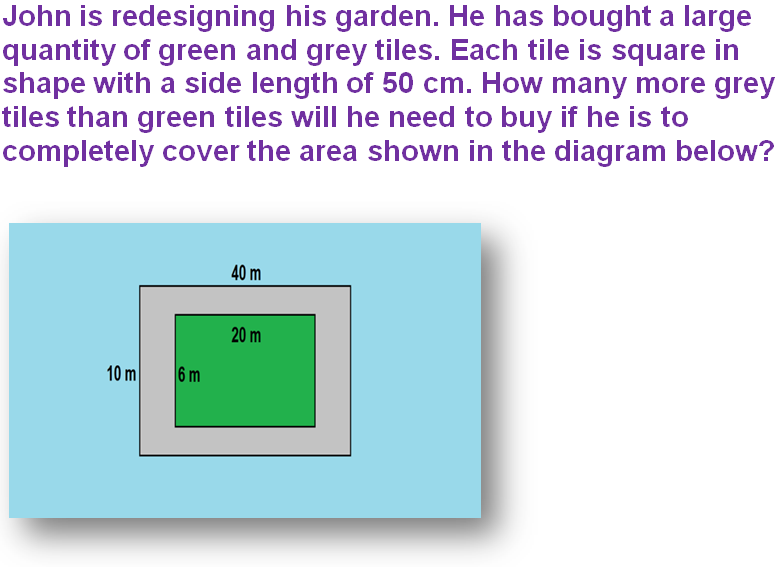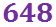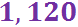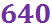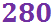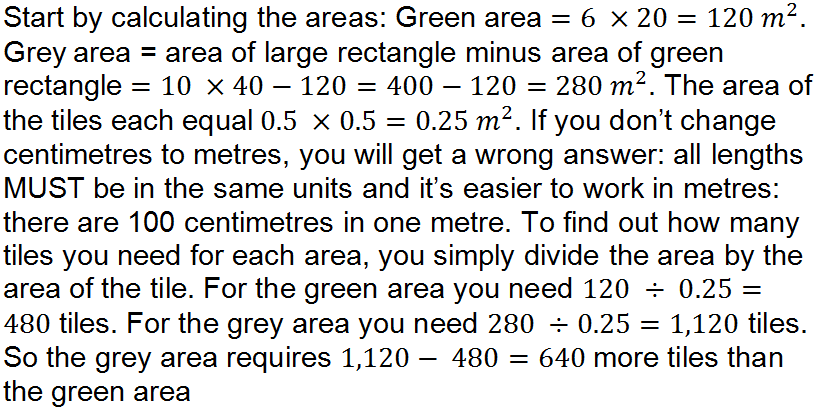Author:  Frank Evans